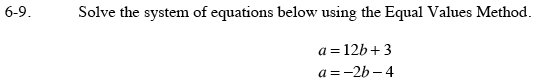### Home > CAAC > Chapter 6 > Lesson 6.1.1 > Problem6-9

6-9.Set the two equations equal to each other.

Solve for b.

12b + 3 = −2b − 4

14b = −7

$b= -\frac{1}{2}$

Substitute b back into one of the original equations to find a.

Remember to check by substituting a and b back into both of the original equations.

$a= 12\left(-\frac{1}{2}\right)+3$

a = −6 + 3

a = −3